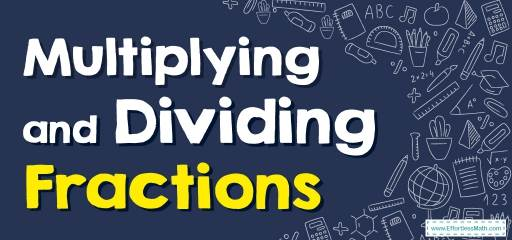# How to Multiply and Divide Fractions? (+FREE Worksheet!)

Multiplying fractions is easier than adding and subtracting them. It only has two simple steps and that's it! To learn it join us.Multiplying fractions may be easier for you than multiplying integers because the numbers you work with in fractions are usually smaller. The other good news is that dividing fractions is as simple as multiplying them. Use the following easy steps to multiply or divide fractions.

## Step-by-step guide to multiplying and dividing fractions

Multiplying fractions:

• Step 1: Multiply the numerators of the fractions by each other to get the numerator of the new fraction.
• Step 2: Multiply the denominators of the fractions by each other to get the denominator of the new fraction.
• Tip: Sometimes when multiplying fractions, you have the opportunity to simplify fractions. For example, when the numerator and denominator of a fraction are both even numbers, this fraction can be simplified. When multiplying fractions, you can usually simplify the fraction by removing equal factors in the numerator and denominator of the fraction. Crossing out equal factors will make the numbers smaller for you, making it easier to work with those numbers. You also get rid of simplifying the fraction at the end of the multiplication.

How to simplify fractions during multiplication operations:

• Step 1: If the numerator of one fraction and the denominator of another fraction are the same number, change both of these numbers to $$1$$ and cross out the corresponding numbers.
• Step 2: When the numerator of one fraction and the denominator of another fraction are both divisible by the same number, factor that number from both (both the numerator and the denominator). In other words, divide the numerator and the denominator by that common factor.

The Greatest Math for Grade 5 Resource

Dividing fractions:

You know that inverse division is multiplication. So to divide fractions:

• Step 1: Write the first fraction in the same way as the original
• Step 2: The division sign is converted to a multiplication sign
• Step 3: The second fraction is reversed and flipped the numerator and denominator
• Step 4: Now you can solve like multiplication of fractions

### Multiplying and Dividing Fractions – Example 1:

Multiply fractions. $$\frac{2}{5} \times \frac{3}{4}=$$

Solution:

Multiply the top numbers and multiply the bottom numbers.
$$\frac{2}{5} \times \frac{3}{4}= \frac{2 \ \times \ 3}{5 \ \times \ 4}=\frac{6}{20}$$ , simplify: $$\frac{6}{20} = \frac{6 \ \div \ 2}{20 \ \div \ 2}=\frac{3}{10}$$

### Multiplying and Dividing Fractions – Example 2:

Divide fractions. $$\frac{1}{2} \div \frac{3}{5}=$$

Solution:

Keep the first fraction, change the division sign to multiplication, and flip the numerator and denominator of the second fraction. Then: $$\frac{1}{2} \times \frac{5}{3} = \frac{1 \ \times \ 5}{2 \ \times \ 3}=\frac{5}{6}$$

### Multiplying and Dividing Fractions – Example 3:

Multiply fractions. $$\frac{5}{6} \times \frac{3}{4}=$$

Solution:

Multiply the top numbers and multiply the bottom numbers.
$$\frac{5}{6}×\frac{3}{4}=\frac{5×3}{6×4}=\frac{15}{24}$$, simplify: $$\frac{15}{24}=\frac{15÷3}{24÷3}=\frac{5}{8}$$

The Absolute Best Book for 5th Grade Students

### Multiplying and Dividing Fractions – Example 4:

Divide fractions. $$\frac{1}{4} \div \frac{2}{3}=$$

Solution:

Keep the first fraction, change the division sign to multiplication, and flip the numerator and denominator of the second fraction. Then: $$\frac{1}{4}×\frac{3}{2}=\frac{1×3}{4×2}=\frac{3}{8 }$$

## Exercises for Multiplying and Dividing Fractions

### Calculate. Simplify if necessary.

1. $$\color{blue}{\frac{1}{5} \times \frac{2}{3}}$$
2. $$\color{blue}{\frac{3}{4} \times \frac{2}{3}}$$
3. $$\color{blue}{\frac{2}{5} \times \frac{3}{7}}$$
4. $$\color{blue}{\frac{2}{9} \div \frac{1}{4}}$$
5. $$\color{blue}{\frac{1}{2} \div \frac{1}{3}}$$
6. $$\color{blue}{\frac{6}{11} \div \frac{3}{4}}$$

1. $$\color{blue}{\frac{2}{15}}$$
2. $$\color{blue}{\frac{1}{2}}$$
3. $$\color{blue}{\frac{6}{35}}$$
4. $$\color{blue}{\frac{8}{9}}$$
5. $$\color{blue}{\frac{3}{2}}$$
6. $$\color{blue}{\frac{8}{11}}$$

The Best Math Books for Elementary Students

### What people say about "How to Multiply and Divide Fractions? (+FREE Worksheet!) - Effortless Math: We Help Students Learn to LOVE Mathematics"?

No one replied yet.

X
51% OFF

Limited time only!

Save Over 51%

SAVE $15 It was$29.99 now it is \$14.99